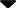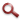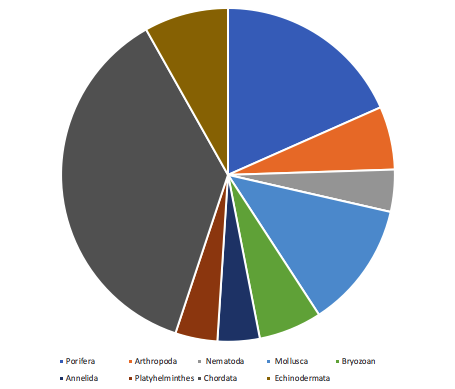Site WebYou are here:

## Student Project FiguresFigure 5 Figure 5: Chart showing the composition of the 2020 plates by phyla. Chordata = 37%, Echinodermata = 8%, Porifera = 18%, Arthropod = 6%, Nematoda = 4%, Mollusca = 12%, Bryozoan = 6%, Annelida = 4%, Platyhelminthes = 4%. Figure 3 Figure 4 Figure 5 Figure 6 Figure 7